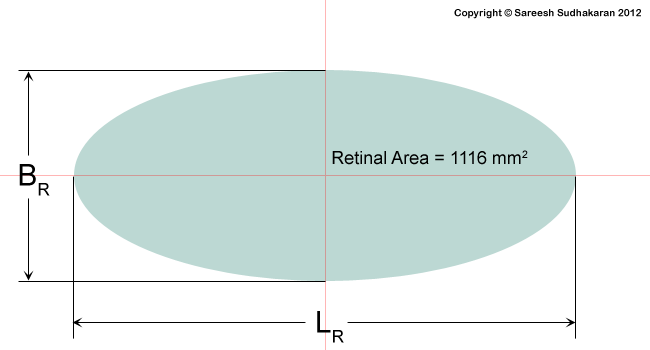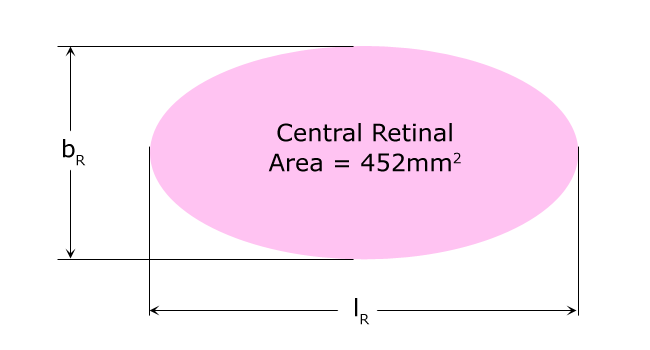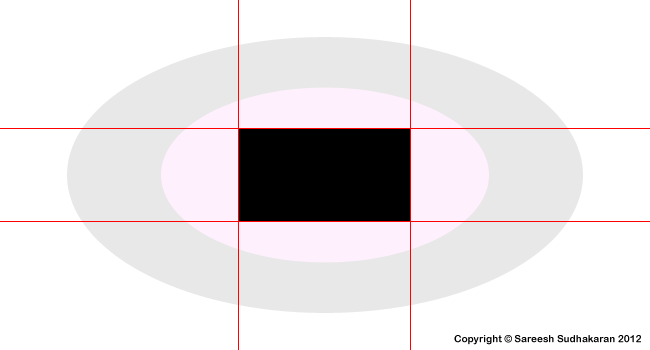Categories

# Notes by Dr. Optoglass: The Megapixel Counts of the Human Eye

Topics Covered:

• The Megapixel Count of the Human Eye
• Rule of Thirds?

Whenever I arrive on a real location, I have to move around and work out what the best angles are going to be. When I was moving around with the lens, I discovered things that the naked eye would not have – Pedro AlmodovarMegapixels by definition assume a fixed area. A 35mm sensor has a fixed size, and the only way to increase megapixels is to reduce the size of each pixel. The eye has a fixed ‘pixel’ size, as I showed earlier.

Depending on the area you define, the megapixel count will change. With that in mind, let’s have some fun.

Absolute Count

We already know the eye has only about a 150 million rods and cones. That’s 150MP right there.

But with a catch. This count is spread over an area of 1116 mm2. A 35mm sensor has an area of 36×24 = 864 mm2. If we take this ratio we are left with 116 MP. But is this enough?

Foveal Count

If the average pixel size of a cone in the fovea is 2 microns and the maximum density of cones in the fovea is 350,000 per mm2, then the total count assuming a 35mm sensor would be 350,000 x 864 = 302.4 MP.

Angle of View Count

If the horizontal angle of view is 155o, and the vertical angle of view is 135o; and the resolution is 0.4 arc minute to 1 arc minute, the number of pixels are:

@ 0.4 arc minute
Horizontal pixel count = (155 / 0.4) x 60 = 23,250
Vertical pixel count = (135 / 0.4) x 60 = 20,250

Total count = 470 Megapixels

@ 1 arc minute =
Horizontal pixel count = (155 / 1) x 60 = 9,300
Vertical pixel count = (135 / 1) x 60 = 8,100

Total count = 75 Megapixels

Wrap-around Count

What if the eye had to be wrapped, just like how we see in the real world? Let’s assume for fun’s sake that the horizontal and vertical angle of view is 200o.

@ 0.4 arc minute =
Horizontal pixel count = (200 / 0.4) x 60 = 30,000
Vertical pixel count = (200 / 0.4) x 60 = 30,000

Total count = 900 MP or about 1 Gigapixel

Take your pick then, from 75 MP to 1 Gigapixel!

The rule of thirds

This is pure conjecture on my part. Take a look at these images:Grey is the full retina. Pink is the central retinal area. If I overlap these, I get this:The red lines are were the 1/3rd lines fall on.

Could it be that, when the eye is fixed, whenever an object travels from an area that falls under the outer retinal area to the central retinal area, more photoreceptor cells are excited, and this change makes this region of particular interest to photographers? Could it be that this ‘change in excitation’ forces the eye to look at these regions on impulse?

Could this be the basis for the famous rule of thirds? You decide.

Takeaways:

• The megapixel count of the eye can be said to be anywhere between 75 MP to 1 Gigapixel.
• Curiously, the ‘rule of thirds’ region falls where the central retinal area begins on the retina.